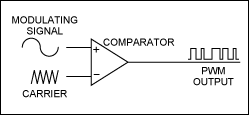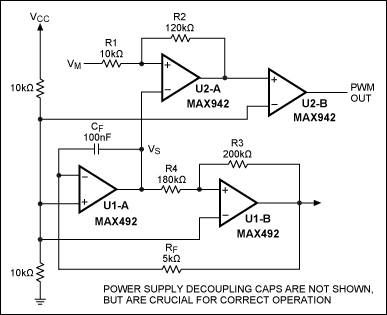# PWM Outputs Enhance Sensor Signal Conditioners

### Abstract

This application note explains that piezoresistive sensors have nonlinear error over temperature. This error can be reduced to within 1% of the sensor's inherent repeatability limitations by using a Maxim sensor signal conditioner and transmitter to transmit sensor information. One transmission method modulates the ratiometric output using pulse-width modulation (PWM). Like its current-loop counterpart, the PWM output has good noise immunity and is suitable for transmission over longer distances. The MAX1452 sensor signal conditioner is featured.

A similar version of this article appeared in the March 2002 issue of Medical Design News magazine.

### Introduction

Most of today's silicon piezoresistive sensors are used in pressure-sensing applications. Fabricated using bulk micro-machining techniques, they are usually arranged as a four-resistor Wheatstone bridge on a single monolithic die. Like integrated circuits, the sensors are inexpensive because they are processed on a wafer containing hundreds to several thousands of sensing elements. Thus far, most of these IC sensors are used for the low- to medium-accuracy requirements of automotive, consumer, and industrial applications.

Piezoresistive sensors are known to have nonlinear error over temperature, which has limited their use in the higher-end markets currently addressed by more costly strain gauges and other sensor types. Correcting the complex errors of a silicon piezoresistive sensor normally requires a considerable amount of electronics, but sensor signal conditioners from Maxim (the MAX1450, MAX1452, MAX1455, MAX1462, and MAX1464) correct these errors to within 1% of the sensor's inherent repeatability limitations. Maxim's sensor signal conditioners create inexpensive transducers, providing total errors < 1% over a wide operating temperature range.

### Benefits of a Current-Loop Transducer

The most common transducer outputs are the ratiometric output (0.5V to 4.5V) and the current-loop output (4mA to 20mA). A standard ratiometric circuit compensates the sensor over the entire temperature range, to an offset of 0.5V and a span of 4.0V. Current loops, for which the desired offset is 4mA and the desired span is 16mA, offer several advantages over the voltage-output transducer. Current loops do not require precise and stable supply voltages; their insensitivity to V = IR drops makes them suitable for long-distance transmission; and the 2-wire twisted pair commonly used for current loops offers very good noise immunity. Power is furnished remotely, through the 4mA of current not needed for information transfer. The 4mA offset also provides a distinction between zero (4mA) and no information (no current flow).

### Pulse-Width Modulation (PWM) Offers Better Operating Efficiency

Another way to transmit sensor information is to modulate the ratiometric output using pulse-width modulation (PWM). The modulating signal (VM) is the transducer's ratiometric output. In a PWM configuration the desired offset corresponds to a 10% duty cycle and the span corresponds to an 80% duty cycle. As the sensor's ratiometric output changes from 0.5V to 4.5V, the corresponding duty cycle changes from 10% to 90%. Like its current-loop counterpart, the PWM output has good noise immunity and is suitable for transmission over longer distances.

PWM provides better operating efficiency in battery-powered applications. In a 5V system the current-loop transducer requires 20mW to 100mW for information transfer. Transmitting the ratio metric output with PWM will require less than 1mW when the PWM transmitter is connected to a high-impedance node.

The simplest way to generate a PWM signal is to compare the message signal to a triangular or ramp waveform. The resulting output is high when the input is greater than the triangular waveform (the carrier), and low when the input is less (Figure 1). Thus, the two basic blocks required for PWM are a triangular-waveform generator and a comparator.Figure 1. A comparator configured as shown produces a PWM output.

In Figure 2, the MAX492 op amp generates the triangular waveform and the MAX942 comparator produces the PWM output signal. Waveforms from this circuit and the relevant equations are shown in Figure 3.Figure 2. The triangular wave in this basic configuration is produced by a dual op amp (U1). The PWM signal is produced by a dual comparator (U2).Figure 3. These waveforms and equations illustrate operation of the circuit in Figure 2.

The MAX492 dual rail-to-rail op amp generates the triangular wave. Rail-to-rail capability is required because the dynamic range of the triangular waveform must be larger than that of the sensor. The integrator circuit (U1-A) generates a positive- or negative-going ramp with slope determined by RF and CF. The comparator stage (U1-B) reverses the slope of the ramp, and the triangular-waveform frequency is given by the equation in Figure 3. For the component values shown, the ramp frequency and thus the PWM output is about 500Hz. Though not shown in Figure 2 and Figure 4, 0.1µF decoupling capacitors should be included at the supply terminals.

A dual comparator (the MAX942) compares the ramp waveform with the sensor's analog voltage. This comparator has the rail-to-rail input range necessary for the application. R1 and R2 provide hysteresis, which ensures accuracy for the PWM output in the presence of rapidly changing sensor inputs. U2-A provides the PWM output, and U2-B provides isolation and additional buffering.

In the complete PWM transducer circuit (Figure 4), the MAX1452 signal conditioner generates a ratiometric compensated output for the Wheatstone bridge, which is then converted to a PWM output. As the MAX1452 output changes with pressure, the PWM-output duty cycle changes accordingly. Note that the MAX1452 can be replaced by any of the analog-output, signal-conditioning application-specific ICs (ASICs) mentioned earlier.Figure 4. Excitation voltage for the Wheatstone bridge (above left) is ratiometric with the power supply in this complete PWM transducer circuit.

Sensor compensation requires an accurate measurement of the PWM output's pulse width, and one instrument for that purpose is a frequency counter with pulse-width-measurement option. You can also interface the PWM output to a microcontroller, and then use the controller's internal timer to calculate the time interval between low-to-high and high-to-low transitions. Either way, the measured PWM value is used to calculate coefficients needed to program the signal-conditioning IC (a MAX1452, for instance).

### Sensor Calibration Using a PWM Output

The MAX1452 incorporates four 16-bit digital-to-analog converters (DACs), a temperature sensor, and temperature-indexed coefficient tables suitable for temperature-compensating and linearizing most bridge sensors. The parameters of offset, span, offset TC, and span TC can be corrected by loading the four corresponding DACs with unique calibration values. Values loaded in the span and offset DACs are derived from a lookup table. When compared with the 2-point compensation algorithm used in the previous generation of signal conditioners, this temperature-driven lookup table adds flexibility and simplifies the calibration process.

Calibration involves loading all four DACs with nominal initial values valid for the transducer family in question. You then set the transducer to temperature T1, and apply maximum pressure. The corresponding PWM output (PWMFS) is measured using one of the methods described above. Next, you measure the PWM output for minimum pressure (PWM0). The PWM span is then PWMSpanT1 = PWMFS -PWM0. Because the required span is normally 80%, the needed span DAC value can be calculated using the expression:

IdealSpanDACT1 = CurrentSpanDAC × 80%/PWMSpanT1
The offset DAC is then set to obtain an output with 10% duty cycle.

To obtain values for the span and offset DACs, repeat the above procedure with the transducer at temperature T2. You can add more temperature points (up to 114) for higher accuracy. Programming up to 114 independent 16-bit EEPROM locations enables correction in 1.5°C increments over the –40°C to +125°C temperature range. (Correction coefficients loaded into the lookup table are linear interpolations between the values recorded for each DAC at each temperature.) Multipoint capability not only enables a simple first-order sensor compensation, but it lets you match unusual temperature curves as well. Following this procedure for the above circuit compensates the sensor quickly, efficiently, and within 0.2% of its inherent repeatability error.